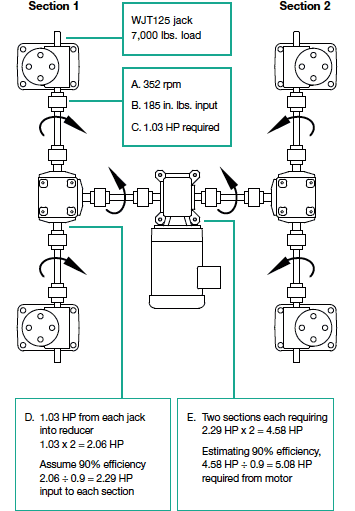# Horsepower Calculation

Operating Torque Constants and Tare Torque values can be found on specification pages. Use the following formula to calculate horsepower:
(RPM x Load (lb) x Operating Torque Constant + Tare Torque)/ 63025 = Horsepower

## Horsepower Example for a Single Jack (WJT242)

WJT242 has a torque constant of 0.009W with (W) representing the load in pounds and a tare torque of 4 inchpounds (page 22). Using 350 RPM on the input shaft and a 2000-pound load results in the following horsepower equation: (350 RPM x 2000 lb x 0.009 + 4 in. lbs) / 63,025 = 0.10 HP

Note: Unlike bevel gear jacks and bevel ball actuators, wormgear style jack input torque requirements vary with input speed, therefore the constants listed in the catalog are only accurate for the RPM listed. To calculate horsepower at speeds other than those listed, please refer to the free JAX® Online Software or fill out a selection guide worksheet and contact Joyce/Dayton.

## Horsepower Example for a 4-Jack System

Find the horsepower required to raise a system load of 28,000-pounds, a distance of 10 inches, at a speed of 11 in./min., using four WJT125 jacks by referencing the Machine Screw Jacks Specifications. The load per jack is 7000 pounds.• A. Determine input speed:
32 turns of the input shaft = 1 inch of linear travel.
(32 turns/inch x 11 inches/min = 352 RPM input)
• B. Determine the input operating torque plus tare torque for one jack:
(0.025 in. lbs. x 7,000) + 10 in. lbs = 185 in. lbs
• C. Determine the input horsepower for one jack:
(352 rpm x 185 in. lbs)/ 63,025 = 1.03 HP per jack

To calculate the horsepower required when operating a jack system, it is usually easiest to separate the system into sections. For example, the "H" system can be viewed as two jack systems joined by a speed reducer in the center.

Always remember to take into account the inefficiencies of miter boxes and gear reducers when calculating system horsepower requirements. (For this exercise use 90% efficiency for miter boxes and gear reducers, but in actual systems efficiencies may differ.)

• D. Determine horsepower required for Section 1:
Total horsepower required for the left side of the system = 1.03 HP per jack x 2 jacks = 2.06 HP

2.06 HP / .9 = 2.29 HP required into miter box of Section 1. Since Sections 1 and 2 are identical, Section 2 also requires 2.29 HP.
• E. Determine horsepower required for Sections 1 and 2:
2.29 HP + 2.29 HP = 4.58 HP

Account for the inefficiency of the central gear reducer to determine the total system horsepower requirement.

4.58 HP / 0.9 = 5.08 HP required to operate this system